In this article, we will share MP Board Class 10th Maths Book Solutions Chapter 7 Coordinate Geometry Ex 7.1 Pdf, These solutions are solved subject experts from the latest edition books.

## MP Board Class 10th Maths Solutions Chapter 7 Coordinate Geometry Ex 7.1

Question 1.
Find the distance between the following pairs of points:
(i) (2, 3), (4, 1)
(ii) (-5, 7), (-1, 3)
(iii) (a, b), (-a, -b)
Solution:
(i) Here x1 = 2, y1 = 3 and x2 = 4, y2 = 1
∴ The required distance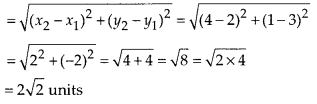(ii) Here x1 = -5, y1 = 7 and x2 = -1, y2 = 3
∴ The required distance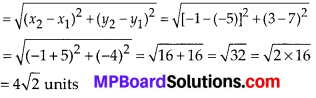(iii) Here x1 = a, y1 = b and x2 = -a, y2 = -b
∴ The required distance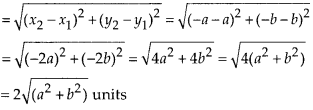Question 2.
Find the distance between the points (0, 0) and (36, 15). Can you now find the distance between the two towns /I and B discussed below as following: ‘A town 6 is located 36 km east and 15 km north of the town A’.
Solution:
Part-I
Let the points be A(0, 0) and B(36, 15)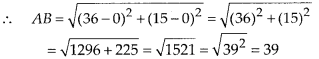Part-II
We have A(0, 0) and B(36,15) as the positions of two towns.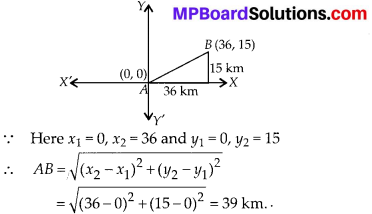Question 3.
Determine if the points (1, 5), (2, 3) and (-2, -11) are collinear.
Solution:
Let the points be A( 1, 5), B(2, 3) and C(-2, -11). A, B and C are collinear, if
AB + BC = AC or AC + CB = AB or BA + AC = BC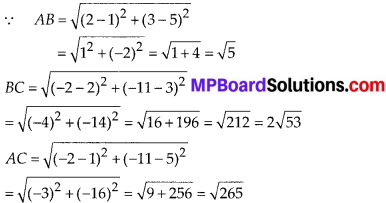Here, AB + BC ≠ AC, AC + CB ≠ AB, BA + AC ≠ BC
∴ A, B and C are not collinear.

Question 4.
Check whether (5, -2), (6, 4) and (7, -2) are the vertices of an isosceles triangle.
Solution:
Let the points be A(5, -2), 6(6, 4) and C(7, -2).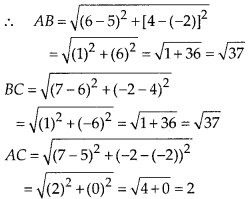We have AB = BC ≠ AC.
∴ ∆ABC is an isosceles triangle.

Question 5.
In a classroom, 4 friends are seated at the points A B, Cand D as shown in the figure. Champa and Chameli walk into the class and after observing for a few minutes Champa asks Chameli, “Don’t you think ABCD is a square?” Chameli disagrees. Using distance formula, find which of them is correct.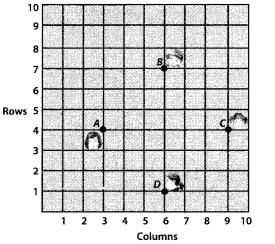Solution:
Let the horizontal columns represent the x-coordinates whereas the vertical rows represent the y-coordinates.
∴ The points are A(3, 4), B(6, 7), C(9, 4) and D(6, 1)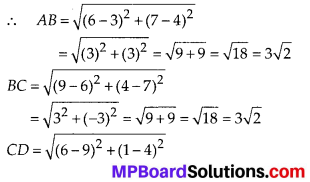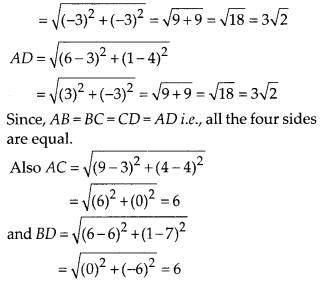i.e., BD = AC ⇒ Both the diagonals are also equal.
∴ ABCD is a square.
Thus, Champa is correct.Question 6.
Name the type of quadrilateral formed if any, by the following points, and give reasons for your answer:
(i) (-1, -2), (1, 0), (-1, 2), (-3, 0)
(ii) (-3, 5), (3, 1), (0, 3), (-1, -4)
(iii) (4, 5), (7, 6), (4, 3), (1, 2)
Solution:
(i) Let the points be A(-1, -2), B(1, 0), C(-1, 2) and D(-3, 0) of a quadrilateral ABCD.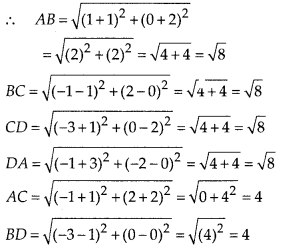⇒ AB = BC = CD = AD i.e., all the sides are equal.
Also, AC and BD (the diagonals) are equal.
∴ ABCD is a square.

(ii) Let the points be A(-3, 5), B(3, 1), C(0, 3) and D(-1, -4).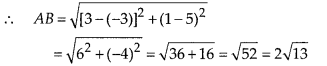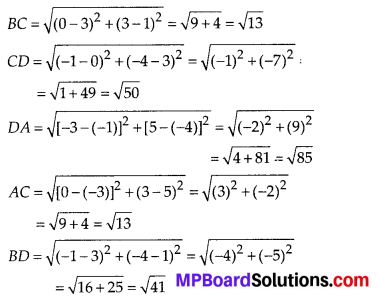We see that $$\sqrt{13}+\sqrt{13}=2 \sqrt{13}$$
i. e., AC + BC = AB
⇒ A, B and C are collinear. Thus, ABCD is not a quadrilateral.

(iii) Let the points be A(4, 5), B(7, 6), C(4, 3) and D(1, 2).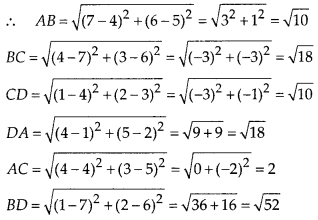Since, AB = CD, BC = DA i.e., opposite sides of the quadrilateral are equal.
And AC ≠ BD ⇒ Diagonals are unequal.
∴ ABCD is a parallelogram.

Question 7.
Find the point on x-axis which is equidistant from (2, -5) and (-2, 9).
Solution:
We know that any point on x-axis has its ordinate = 0
Let the required point be P(x, 0)
Let the given points be A(2, -5) and B(-2, 9)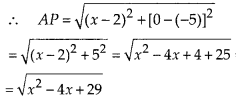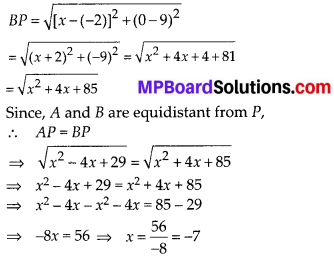∴ The required point is (-7, 0)

Question 8.
Find the values of y for which the distance between the points P(2, -3) and Q(10, y) is 10 units.
Solution:
The given points are P(2, -3) and Q(10, y).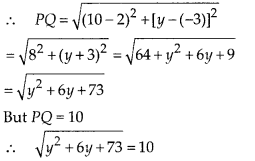Squaring both sides, y2 + 6y + 73 = 100
⇒ y2 + 6y – 27 = 0
⇒ y2 – 3y + 9y – 27 = 0
⇒ (y – 3)(y + 9) = 0
Either y – 3 = 0 ⇒ y = 3
or y + 9 = 0 ⇒ y = -9
∴ The required value of y is 3 or -9.Question 9.
If Q(0, 1) is equidistant from P(5, -3) and R(x, 6), find the values of x. Also find the distances QR and PR.
Solution: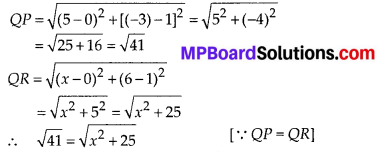Squaring both sides, we have x2 + 25 = 41
⇒ x2 + 25 – 41 = 0
⇒ x2 – 16 = 0 2 x = $$\pm \sqrt{16}$$ = ±4
Thus, the point R is (4, 6) or (-4, 6)
Now,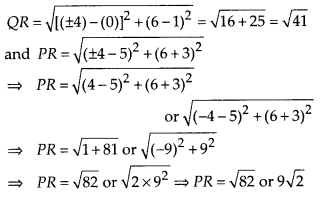Question 10.
Find a relation between x and y such that the point (x, y) is equidistant from the point (3, 6) and (-3, 4).
Solution:
Let the points be A(x, y), B(3, 6) and C(-3, 4).
∴ AB = $$\sqrt{(3-x)^{2}+(6-y)^{2}}$$
And AC = $$\sqrt{[(-3)-x]^{2}+(4-y)^{2}}$$
Since, the point (x, y) is equidistant from (3, 6) and (-3, 4).
∴ AB = AC
⇒ $$\sqrt{(3-x)^{2}+(6-y)^{2}}=\sqrt{(-3-x)^{2}+(4-y)^{2}}$$
Squaring both sides,
(3 – x)2 + (6 – y)2 = (-3 – x)2 + (4 – y)2
⇒ (9 + x2 – 6x) + (36 + y2 – 12y)
⇒ (9 + x2 + 6x) + (16 + y2 – 8y)
⇒ 9 + x2 – 6x + 36 + y2 – 12y – 9 – x2 – 6x – 16 – y2 + 8y = 0
⇒ – 6x – 6x + 36 – 12y – 16 + 8y = 0
⇒ – 12x – 4y + 20 = 0
⇒ -3x – y + 5 = 0
⇒ 3x + y – 5 = 0 which is the required relation between x and y.